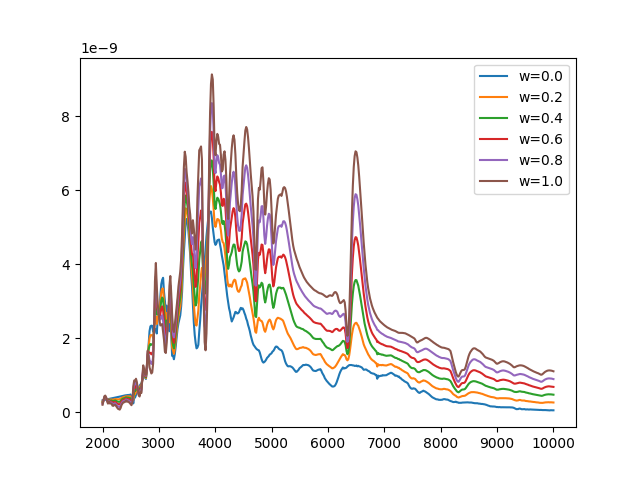# Creating a new Source class¶

Extending sncosmo with a custom type of Source.

A `Source` is something that specifies a spectral timeseries as a function of an arbitrary number of parameters. For example, the SALT2 model has three parameters (`x0`, `x1` and `c`) that determine a unique spectrum as a function of phase. The `SALT2Source` class implements the behavior of the model: how the spectral time series depends on those parameters.

If you have a spectral timeseries model that follows the behavior of one of the existing classes, such as `TimeSeriesSource`, great! There’s no need to write a custom class. However, suppose you want to implement a model that has some new parameterization. In this case, you need a new class that implements the behavior.

In this example, we implement a new type of source model. Our model is a linear combination of two spectral time series, with a parameter `w` that determines the relative weight of the models.

```import numpy as np
from scipy.interpolate import RectBivariateSpline
import sncosmo

class ComboSource(sncosmo.Source):

_param_names = ['amplitude', 'w']
param_names_latex = ['A', 'w']   # used in plotting display

def __init__(self, phase, wave, flux1, flux2, name=None, version=None):
self.name = name
self.version = version
self._phase = phase
self._wave = wave

# ensure that fluxes are on the same scale
flux2 = flux1.max() / flux2.max() * flux2

self._model_flux1 = RectBivariateSpline(phase, wave, flux1, kx=3, ky=3)
self._model_flux2 = RectBivariateSpline(phase, wave, flux2, kx=3, ky=3)
self._parameters = np.array([1., 0.5])  # initial parameters

def _flux(self, phase, wave):
amplitude, w = self._parameters
return amplitude * ((1.0 - w) * self._model_flux1(phase, wave) +
w * self._model_flux2(phase, wave))
```

… and that’s all that we need to define!: A couple class attributes (`_param_names` and `param_names_latex`, an `__init__` method, and a `_flux` method. The `_flux` method is guaranteed to be passed numpy arrays for phase and wavelength.

We can now initialize an instance of this source from two spectral time series:

```#Just as an example, we'll use some undocumented functionality in
# sncosmo to download the Nugent Ia and 2p templates. Don't rely on this
# the `DATADIR` object, or these paths in your code though, as these are
# subject to change between version of sncosmo!

# In our __init__ method we defined above, the two fluxes need to be on
# the same grid, so interpolate the second onto the first:
flux2_interp = RectBivariateSpline(phase2, wave2, flux2)(phase1, wave1)

source = ComboSource(phase1, wave1, flux1, flux2_interp, name='sn1a+sn2p')
```
```Downloading http://c3.lbl.gov/nugent/templates/sn1a_flux.v1.2.dat.gz [Done]
```

We can get a summary of the Source we created:

```print(source)
```
```class      : ComboSource
name       : 'sn1a+sn2p'
version    : None
phases     : [0, .., 90] days
wavelengths: [1000, .., 25000] Angstroms
parameters:
amplitude = 1.0
w         = 0.5
```

Get a spectrum at phase 10 for different parameters:

```from matplotlib import pyplot as plt

wave = np.linspace(2000.0, 10000.0, 500)
for w in (0.0, 0.2, 0.4, 0.6, 0.8, 1.0):
source.set(w=w)
plt.plot(wave, source.flux(10., wave), label='w={:3.1f}'.format(w))

plt.legend()
plt.show()
```The w=0 spectrum is that of the Ia model, the w=1 spectrum is that of the IIp model, while intermediate spectra are weighted combinations.

We can even fit the model to some data!

```model = sncosmo.Model(source=source)
result, fitted_model = sncosmo.fit_lc(data, model,
['z', 't0', 'amplitude', 'w'],
bounds={'z': (0.2, 1.0),
'w': (0.0, 1.0)})

sncosmo.plot_lc(data, model=fitted_model, errors=result.errors)
``````<Figure size 780x670 with 8 Axes>
```

The fact that the fitted value of w is closer to 0 than 1 indicates that the light curve looks more like the Ia template than the IIp template. This is generally what we expected since the example data here was generated from a Ia template (although not the Nugent template!).

Total running time of the script: ( 0 minutes 3.145 seconds)

Gallery generated by Sphinx-Gallery